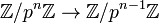# Quasicyclic group

Jump to: navigation, search
This article is about a family of groups with a parameter that is prime. For any fixed value of the prime, we get a particular group.
View other such prime-parametrized groups

## Definition

Let$p$ be a prime number. The$p$-quasicyclic group is defined in the following equivalent ways:

• It is the group, under multiplication, of all complex$(p^n)^{th}$ roots of unity for all$n$.
• It is the quotient$L/\mathbb{Z}$ where$L$ is the group of all rational numbers that can be expressed with denominator a power of$p$.
• It is the direct limit of the chain of groups:$\mathbb{Z}/p\mathbb{Z} \to \mathbb{Z}/p^2\mathbb{Z} \to \dots \to \mathbb{Z}/p^n\mathbb{Z} \to$.

where the maps are multiplication by$p$ maps.

## Particular cases

Prime number$p$$p$-quasicyclic group
2 2-quasicyclic group
3 3-quasicyclic group

## Group properties

Property Satisfied? Explanation Corollary properties satisfied
abelian group Yes Hence, it is also a nilpotent group and a solvable group.
locally cyclic group Yes
locally finite group Yes
p-group Yes Hence, it is an abelian p-group, so also a nilpotent p-group.

## Related notions

### Combining quasicyclic groups for all primes

The restricted external direct product of the$p$-quasicyclic groups for all prime numbers$p$ is isomorphic to$\mathbb{Q}/\mathbb{Z}$, the group of rationals modulo integers.

### p-adics: inverse limit instead of direct limit

The additive group of p-adic integers can, in a vague sense, be considered to be constructed using a method dual to the method used to the quasicyclic group. While the$p$-adics are constructed as an inverse limit for surjective maps$\mathbb{Z}/p^n\mathbb{Z} \to \mathbb{Z}/p^{n-1}\mathbb{Z}$, the quasicyclic group is constructed as a direct limit for injective maps$\mathbb{Z}/p^{n-1}\mathbb{Z} \to \mathbb{Z}/p^n\mathbb{Z}$.